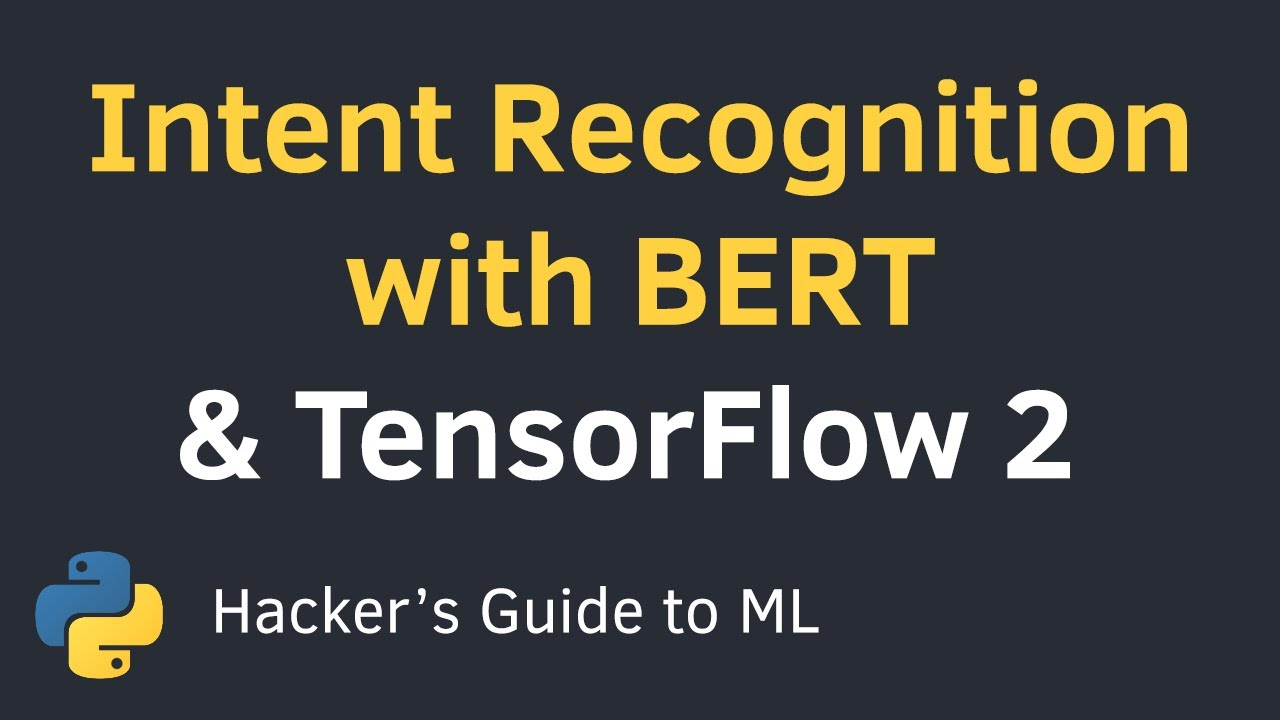# Intent Recognition with BERT using Keras and TensorFlow 2 in Python | Text Classification TutorialLearn how to fine-tune the BERT model for text classification. Train and evaluate it on a small dataset for detecting seven intents. The results might surprise you!

Learn how to fine-tune the BERT model for text classification. Train and evaluate it on a small dataset for detecting seven intents. The results might surprise you!

## top 30 Python Tips and Tricks for Beginners

In this post, we'll learn top 30 Python Tips and Tricks for Beginners

## Lambda, Map, Filter functions in python

You can learn how to use Lambda,Map,Filter function in python with Advance code examples. Please read this article

## Keras vs. Tensorflow - Difference Between Tensorflow and Keras

Keras vs Tensorflow - Learn the differences between Keras and Tensorflow on basis of Ease to use, Fast development,Functionality,flexibility,Performance etc

## Pytorch vs Tensorflow vs Keras | Deep Learning Tutorial (Tensorflow, Keras & Python)

We will go over what is the difference between pytorch, tensorflow and keras in this video. Pytorch and Tensorflow are two most popular deep learning frameworks. Pytorch is by facebook and Tensorflow is by Google. Keras is not a full fledge deep learning framework, it is just a wrapper around Tensorflow that provides some convenient APIs.

## Word Embedding using Keras Embedding Layer | Deep Learning Tutorial 40 (Tensorflow, Keras & Python)

Word Embedding using Keras Embedding Layer | Deep Learning Tutorial (Tensorflow, Keras & Python) | We will discuss how exactly word embeddings are computed. There are two techniques for this (1) supervised learning (2) self supervised learning techniques such as word2vec, glove. In this tutorial we will look at the first technique of supervised learning. We will also write code for food review classification and see how word embeddings are calculated while solving that problem